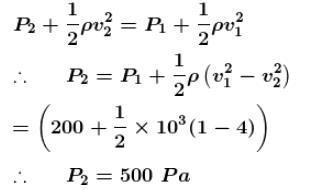Courses

# Fluid Dynamics NAT Level - 1

## 10 Questions MCQ Test Basic Physics for IIT JAM | Fluid Dynamics NAT Level - 1

Description
This mock test of Fluid Dynamics NAT Level - 1 for Physics helps you for every Physics entrance exam. This contains 10 Multiple Choice Questions for Physics Fluid Dynamics NAT Level - 1 (mcq) to study with solutions a complete question bank. The solved questions answers in this Fluid Dynamics NAT Level - 1 quiz give you a good mix of easy questions and tough questions. Physics students definitely take this Fluid Dynamics NAT Level - 1 exercise for a better result in the exam. You can find other Fluid Dynamics NAT Level - 1 extra questions, long questions & short questions for Physics on EduRev as well by searching above.
*Answer can only contain numeric values
QUESTION: 1

### An open tank 10m long and 2m deep is filled up to 1.5m  height of oil of specific gravity 0.82. The tank is uniformly accelerated along its length from rest to a speed of  20 m/sec  horizontally. The shortest time (in sec) in which the speed may be attained without spilling any oil is: g=10 m/sec2

Solution: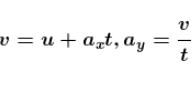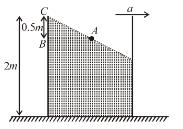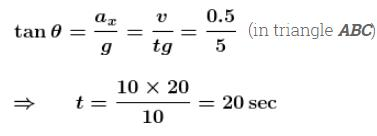*Answer can only contain numeric values
QUESTION: 2

### An open water tanker moving on a horizontal straight road has a cubical block of cork floating over its surface. If the tanker has an acceleration of a as shown, the acceleration (in  m/s2) of the cork w.r.t. container is (ignore viscosity)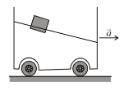Solution: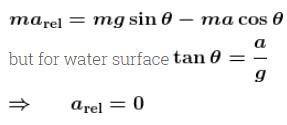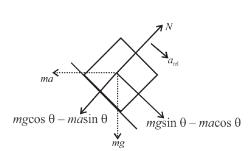*Answer can only contain numeric values
QUESTION: 3

### A fire hydrant delivers water of density ρ at a volume rate  L. The water travels vertically upward through the hydrant and then does 90° turn to emerge horizontally at speed  V.  The pipe and nozzle have uniform cross section throughout. The force exerted by the water on the corner of the hydrant is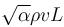.  Find the value of  α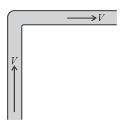Solution:

Force exerted in vertical direction and horizontal direction are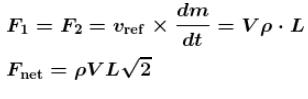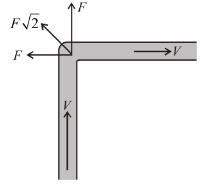*Answer can only contain numeric values
QUESTION: 4

Water is moving with a speed of  5.18 m/s–1  through a pipe with a cross-sectional area of  4.20 cm–2.  The water gradually descends 9.66m  as the pipe increase in area to 7.60cm2. The speed (in  ms–1) of flow at the lower level is :

Solution: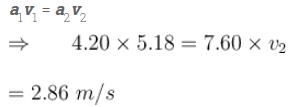*Answer can only contain numeric values
QUESTION: 5

An incompressible liquid flows through a horizontal tube as shown in the figure. Then the velocity v (in m/s) of the fluid is :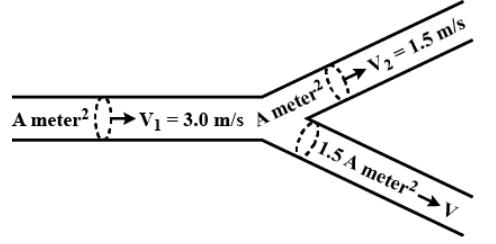Solution: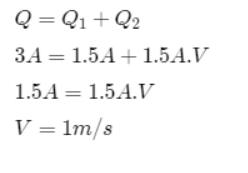*Answer can only contain numeric values
QUESTION: 6

Assuming the xylem tissues through which water rises from root to the branches in a tree to be of uniform cross-section. Find the maximum radius (in  mu m) of xylem tube in a 10m high coconut tree so that water can rise to the top.
(Surface tension of water = 0.1N/m  Angle of contact of water with xylem tube = 60°)

Solution: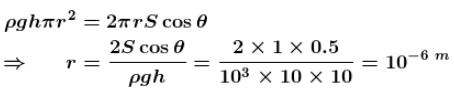*Answer can only contain numeric values
QUESTION: 7

A tank is filled with a liquid upto a height H. A small hole is made at the bottom of this tank. Let  t1  be the time taken to empty first half of the tank t2 the time taken to empty rest half of the tank. Then find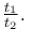Solution:

Substituting the proper limits in Equation (1), derived in the theory, we have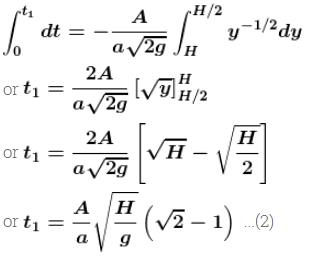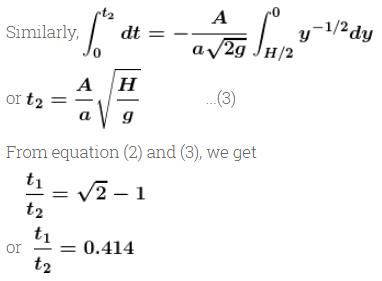*Answer can only contain numeric values
QUESTION: 8

A large open tank has two small holes in its vertical wall as shown in figure. One is a square hole of side l at a depth 4y from the top and the other is a circular hole of radius R at a depth y from the top. When the tank is completely filled with water, the quantities of water flowing out per second from both holes are the same. Then R is equal to  αL. Find the value of  α.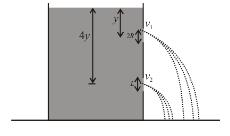Solution: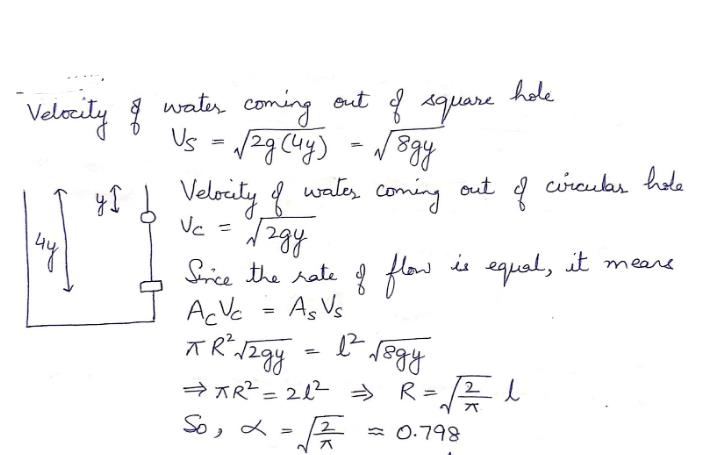*Answer can only contain numeric values
QUESTION: 9

The velocity of the liquid coming out of a small hole of a large vessel containing two different liquids of densities 2ρ and ρ as shown in figure is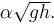Find the value of  α.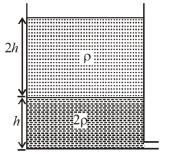Solution:

Pressure at (1) :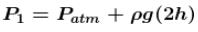Applying Bernoulli’s theorem between points (1) and (2)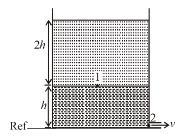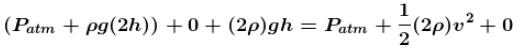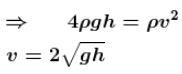*Answer can only contain numeric values
QUESTION: 10

A horizontal pipe line carries in a streamline flow. At a point along the pipe where the cross-sectional area is 10 cm2, the water velocity is 1ms–1 and the pressure is 2000 Pa. What will be the pressure (in Pa) of water at another point where the cross-sectional area is 5cm2?

Solution:

From continuity equation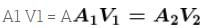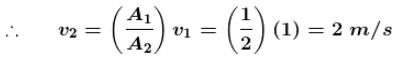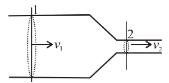Applying Bernoulli’s theorem at 1 and 2